# What are Momentum and Impulse Notes pdf ppt

## What is Momentum?

Momentum is a word that we hear used colloquially in everyday life. We are often told that sports teams and political candidates have “a lot of momentum”. In this context, the speaker usually means to imply that the team or candidate has had a lot of recent success and that it would be difficult for an opponent to change their trajectory. This is also the essence of the meaning in physics, though in physics we need to be much more precise.
Momentum is a measurement of mass in motion: how much mass is in how much motion. It is usually given the symbol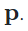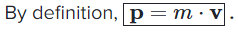Where m is the mass and is the velocity. The standard units for momentum are , and momentum is always a vector quantity. This simple relationship means that doubling either the mass or velocity of an object will simply double the momentum.
The useful thing about momentum is its relationship to force. You might recall from the kinematic equations that change in velocitycan also be written as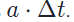We can then see that any change in momentum following an acceleration can be written as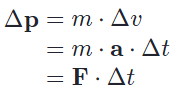## What is Impulse?

Impulse is a term that quantifies the overall effect of a force acting over time. It is conventionally given the symbol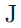and expressed in Newton-seconds.
For a constant force,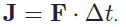As we saw earlier, this is exactly equivalent to a change in momentum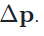. This equivalence is known as the impulse-momentum theorem. Because of the impulse-momentum theorem, we can make a direct connection between how a force acts on an object over time and the motion of the object.
One of the reasons why impulse is important and useful is that in the real world, forces are often not constant. Forces due to things like people and engines tend to build up from zero over time and may vary depending on many factors. Working out the overall effect of all these forces directly would be quite difficult.
When we calculate impulse, we are multiplying force by time. This is equivalent to finding the area under a force-time curve. This is useful because the area can just as easily be found for a complicated shape—variable force—as for a simple rectangle—constant force. It is only the overall net impulse that matters for understanding the motion of an object following an impulse.
The concept of impulse that is both external and internal to a system is also fundamental to understanding conservation of momentum.

## Momentum in Space:

Most people are familiar with seeing astronauts working in orbit. They appear to effortlessly push around freely floating objects. Because astronauts and the objects they are working with are both in free-fall, they do not have to contend with the force of gravity. However, heavy moving objects still posses the same momentum that they do on earth, and it can be just as difficult to change this momentum.
Suppose that an emergency occurs on a space station and an astronaut needs to manually move a free-floating 4,000 kg space capsule away from a docking area. On earth, the astronaut knows she can hold a 50 kg weight above herself for 3 seconds. How quickly could she get the capsule moving?
We first calculate the total impulse that the astronaut can apply. Note that the astronaut is pushing vertically in both cases so we don’t need to keep track of the direction of the force.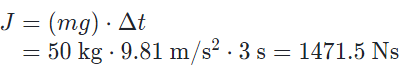And, by the impulse-momentum theorem, we can find the velocity of the spacecraft: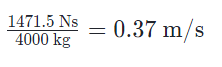## What is Specific Impulse?

Specific impulse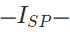start subscript, S, P, end subscript—is a specification commonly given to engines which produce a thrust force. Jet engines and rocket engines are two common examples. In this context, specific impulse is a measure of the efficiency of using fuel to produce thrust and is one of the most important specifications of such an engine.
When the prefix specific is used in physics, it means “relative to” a particular quantity. Specific gravity and specific heat are two examples of where you may have seen this prefix used. Specific impulse is impulse measured relative to the weight of fuel—on earth—used to produce the impulse.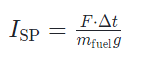Because we are dividing an impulse by a force—the force on the fuel due to earth’s gravity—the force units cancel out, and the units for specific impulse are simply seconds.

### Feedback is important to us.

error: Content is protected !!